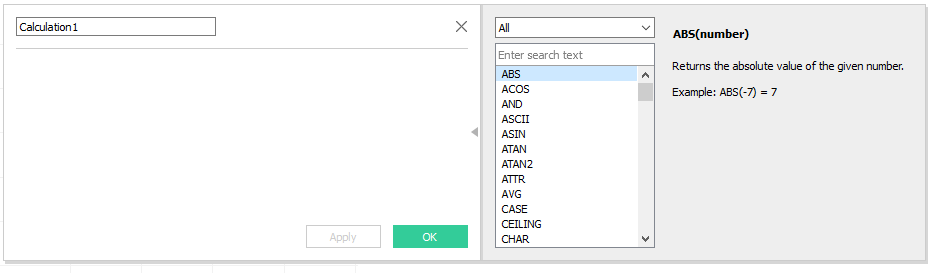# Tableau Calculated Field

A calculated field allows you to do two major functions:

1. Create a New Field based on existing Measures and Dimensions
2.  Alter an Existing Field

What is a calculated field in Tableau

I think it’s only fitting to establish exactly what a field is. A field comprises the the dimension and metrics that are part of the data you have. These are all listed on the data pane to the left. A calculated field is a custom metric or dimension that you create using a calculation or rule. This can range from user, logical, type manipulation, aggregate function,  number functions, and date functions. This is a very useful subject that will allow you to transform your data with built-functions.

How to Create a Calculated Field

• Option 1: Right Click an empty space in the data pane and choose calculated field. At this point you can create a multitude of new fields to help you better understand your data. Open the window is open you will have a formula box and provide you with a list of functions that you can use to create your calculated field.
• Open 2: Right click a dimension or measure field  and select a calculated field based on that element.
• Option 3: Drag the a field into the formula boxTen Common Formulas for Calculated Field

1. Case Function

Case function is similar to an If statement. Case creates a conditions that must be met to return a result. Here is a simple example of a case formula.

CASE [Weekday] WHEN “Monday” THEN “Working” WHEN “Sunday” THEN “Not Working” ELSE “Closed” END

2. IF Function

IF is similar to Case. However, it allows you to mix different data types such as strings and integers.

IF [Profit]>[Cost] THEN “Profitable” ELSE “Not Profitable” END

3.  MAX

This returns the maximum value in a data set.

MAX(1232, 3243,555,90,345) = 3243

4. MIN

This returns the minimum value in a data set.

MIN(1232, 3243,555,90,345) = 90

5. DATEPARSE

This function will convert a string into a date format of your specification.

DATEPARSE(mm.dd.yyyy, “29/July/2001”) = July-29-2001

7. CONTAINS

This is a string function that check whether a string elements exist in another string.

CONTAINS (“Absentdata”, “data”) = TRUE

8. SUM/COUNT/AVERAGE

9. TRIM

This function removes spaces around a string.

TRIM(” Biggy  “) = “Biggy”

10. MEDIAN

Returns the middle number in a sequence of numbers

MEDIAN(13,44,66,90,134,200,250) =90